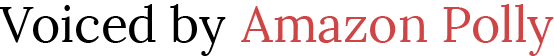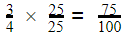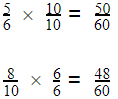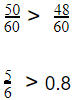# Teaching Fractions and Decimals

 Would You Rather Listen to the Lesson?In pre-algebra units, you’ll be teaching fractions and decimals to students to help them understand what they represent. Students then become proficient in converting fractions to decimals and vice versa, as well as comparing fractions and decimals.

There are many ways in which math teachers can make lessons in fractions and decimals, whether it’s by incorporating games, videos, etc. Without further ado, here are some awesome tips on teaching this topic that will make the class atmosphere as inviting as it gets!

## Tips on Teaching Fractions and Decimals

### What Are Fractions and Decimals?

You can start your lesson by briefly reviewing what fractions and decimals are. Point out that a fraction is a mathematical expression representing the division of one whole number by another. Provide a few examples, such as 3⁄4, 1⁄2, 5⁄8, etc.

Add that, in fractions, the top number is called a numerator, whereas the bottom number is called a denominator. For instance, in 3⁄4, the numerator is 3, and the denominator is 4; in 5⁄8, the numerator is 5, and the denominator is 8, etc.

Then, define a decimal as a number comprising a decimal point, followed by digits whose value is smaller than one. So decimals contain a number part and a fractional part, and these two are separated by a decimal point. Provide examples, such as 0.02, 3.8, 5.1, etc.

### Conversion Between Fractions and Decimals

#### Converting Decimals to Fractions

Explain to students that when we want to convert a decimal to a fraction, we need to re-write the decimal as a fraction that has a denominator with powers of 10 and a numerator as the number without a decimal point.

Add that if the given decimal has n digits after the decimal point, we will rewrite it as a fraction with denominator 10n. Provide a few examples to illustrate how this works in practice.

##### Example 1:

Write a decimal on the whiteboard, such as 0.345, and tell students that we want to convert this decimal to a fraction. First, we will need to choose a denominator with powers of 10. To determine the powers of 10, we will check the number of digits after the decimal point.

In 0.345, we can observe that there are 3 digits after the decimal point, which means that the denominator will be ten to the power of three, that is, 10³. The only thing left to do now is to determine the numerator.

To do this, we look at the whole decimal and we just take the number without the decimal point, i.e. 345:#### Converting Fractions to Decimals

Explain to students that in order to convert a fraction to a decimal, we need to re-write the fraction so the denominator has powers of 10. We do this by multiplying the numerator and denominator by an appropriate number.

• If the numerator is smaller than the denominator, we write the digits in the numerator after the decimal digit. Zeros are added after the decimal if the denominator has more digits than the numerator.
• If the numerator is greater than the denominator, the decimal point is shifted from left to right by the same digits as in the denominator.
##### Example 1:

Write a fraction on the whiteboard, such as 3⁄4, and tell students that we want to convert it to a decimal. First, we need to transform this fraction into one that has a denominator with powers of 10.

So we need to find a number to multiply the current denominator so that the bottom of the new fraction is 10, 100, 1,000, etc. The denominator of the current fraction is 4, so let’s try to rewrite it as 100. To do this, we need to multiply 4 by 25.

Now we also need to multiply the current numerator, that is, 3, by the same number by which we multiplied the denominator so that the value of the fraction remains unchanged. In other words, we also need to multiply 3 by 25.Point out that to finalize the process of conversion, we need to check whether the top number is greater or smaller than the bottom number. Since in this case 75 is smaller than 100, we write the digits in the top number after the decimal digit:

Add that since 100 has two zeros, we will write 75 by placing the decimal point two spaces from the right:

0.75

3⁄4= 0.75

### Comparing Fractions and Decimals

Understanding how to convert fractions to decimals and vice versa is a prerequisite for comparing fractions and decimals. Now that students are fluent in conversions, point out that there are two approaches when it comes to comparing fractions and decimals:

1. We convert the decimals to fractions first, then make the denominators of both fractions the same, and then simply compare the numerators. The fraction with a greater numerator is greater.
2. We convert the fractions to decimals first and then compare the decimals.

#### Example:

Write the 5⁄6 and 0.8 on the whiteboard and tell students that we want to compare the two by using the inequality symbols <, > and =. Point out that we’ll start by converting the decimal 0.8 to a fraction.

By applying the steps that we enumerated above, we’ll first turn the decimal into a fraction with a denominator with powers of 10 and a numerator as the number without a decimal point. That is, 0.8 equals 8⁄10.

Point out that to compare the two fractions, we first need to make their denominators (10 and 6) the same. We need to find the common multiple for that, for example, 60. So we’ll transform the two fractions into two new fractions having 60 as the denominator. In other words:Add that the only thing left to do now that we have the same denominator in both fractions is to compare the different numerators and see which one is bigger. The fraction whose numerator has a greater value is the one that’s greater:If you have the technical means in your classroom, you may also want to include multimedia materials, such as videos in your lesson on converting between fractions and decimals and comparing fractions and decimals.

For example, you can use this video to illustrate the process of converting decimals to fractions, and this one to demonstrate how to convert fractions to decimals. You can also play this video that contains simple guidelines on how to compare fractions and decimals.

You may also want to check out this article that presents three cool methods of converting between fractions, decimals, and percentages, based on real-world scenarios and examples, connected to food, money, and cell phone minutes.

## Activities to Practice Fractions and Decimals### Fractions and Decimals Game

This is an engaging matching game that will help students practice their skills at converting between fractions and decimals. To implement this game in your classroom, you’ll need violet and yellow construction paper, scissors, and some markers.

Use the markers to draw two sets of cards on the construction paper, one set of cards is on the violet paper, and the other set of cards on the yellow paper. For instance, the yellow set of cards can be fractions and the violet one decimals (one fraction and one decimal per card).

For each yellow card that you create, make sure that there is a matching violet card, that is, for each fraction that you write, there should be a matching decimal. For instance, if you write 5⁄6 on a yellow card, make sure there’s a violet card with 0.8 on it.

Divide students into groups of 3 or 4 and hand out one yellow and one violet set of cards to each group. Provide instructions for the game. Tell students that they have to match each yellow card with an equivalent violet card that has the same value as fast as possible.

The cards remain face down until you signal to students that the game can start. The first group to finish matching all cards correctly wins the game. Assign a checker in each group to facilitate the process of checking.

### Fruit Splat! Fractions to Decimals Game

This is a fun online game that will help students hone their ability to convert fractions to decimals. To use this game in your class, make sure there’s a suitable device for every student and a decent internet connection.

The game is played individually, which makes it great for homeschooling parents also. Explain to students that they are assigned a fraction with which they target the fruit decimal with the equivalent value.

If they hit the correct fruit fraction, they will hear a ‘splat’ and score points. If they hit the wrong fruit decimal, they don’t score anything. Keep playing until time allows it. As a bonus, you’ll find that the game is amazing for training the mental math skills of students.

### Death to Decimals and the Adventures of Fraction Man

This is an awesome online game that students are guaranteed to enjoy. The game is useful for helping students sharpen their understanding of fractions and decimals with equivalent values, and how to quickly convert between fractions and decimals.

Students play the game individually. Explain to students that in the game, they are a ‘fraction hero’ that has to save a large city from aliens who are attacking it with decimal points. To save the city, the ‘fraction hero’ has to convert the decimals to safe fractions.

Play the game as long as students enjoy it or as long as time allows it. Afterward, open a discussion and reflect on the game together with the students. Which steps did they use to convert the decimals? Were there any decimals that they struggled with in particular?

## Before You Leave…

If you enjoyed these strategies and activities on teaching fractions and decimals, and you’re looking for more math materials for children of all ages, sign up for our emails and get loads of free lessons and content!

Make sure to also head over to our blog – you’ll find plenty of cool resources for your class!